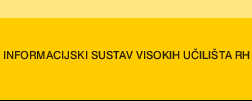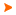Podaci o VU Početna stranicaArhiva nastavnih programaArhiva ak. kalendaraAbbreviation: B11A02 Load: 30(L) + 0(P) + 0(FE) + 0(LE) + 3(S) + 0(PEE) + 27(E) + 0(DE)
 Lecturers in charge: v. pred. mr. sc. Vida Zadelj-Martić Lecturers: Course description: Understanding the key topics and problems of Mathematical Analysis. Also it is necessary to develop many skills between abstract entities according to certain rules and apply it into GeodesyLearning outcomes at the level of the programme to which the course contributesDemonstrate competences in theoretical principles, procedures of computing and visualising the surveying data.Understand mathematical methods and physical laws applied in geodesy and geoinformatics.Apply knowledge of mathematics and physics for the purpose of recognizing, formulating and solving of problems in the field of geodesy and geoinformatics.Exercise appropriate judgements on the basis of performed calculation processing and interpretation of data obtained by means of surveying and its results.Take responsibility for continuing academic development in the field of geodesy and geoinformatics, or related disciplines, and for the development of interest in lifelong learning and further professional education.Learning outcomes expected at the level of the course (4 to 10 learning outcomes)Define and implement the tasks terms of mathematical logic, sets, sets of numbers and mathematical inductionDefine, analyze and relate the concepts and properties of real functions of a real variable, as well as terms related to a sequences (limit of a sequence, limit of a function)Define and apply the concepts tasks derivatives, indefinite and definite integralsDefine and apply the concepts tasks series of numbers, functional series and power series, as a Taylor series expansion and Mac Lauren seriesDefine, analyze and apply the tasks terms of functions of several variables, as well as Taylor and Maclaurin series for two variables, and to determine the extreme values of functions of two variablesDefine the term and solve differential equations method of separation of variablesCourse content broken down in detail by weekly class schedule (syllabus)Mathematical logic and sets; Sets of numbers and mathematical induction (P1h + V1H)Real functions of a real variable (function definition, the term of natural domain, injections, surjective, bijective function of function, monotony, parity and periodicity of functions, composition of functions, inverse function) (P1h + V1H)Elementary functions (polynomial, rational, exponential and logarithmic functions, general power, trigonometric functions, inverse trigonometric, hyperbolic, area functions; properties and graphs) (P2h + V2H)Sequences and limit of s sequence (term series, bounded and monotone sequences, limit of sequence, properties of convergent sequences,) (P1h + V1H)Limit and continuity of functions (limit of function, performance limit of a function, continuity of functions, properties) (P2h + V2H)Derivative and some theorems of differential calculus (speed problem, the problem of tangents, the definition of derivatives, higher order derivatives, differential function, differentiation rules, derivative composition of a function, derivation of elementary functions, logarithmic differentiation, derivative of implicit functions, derivation of a parametric function, equation of tangents and normals at the point in the curve, the equation of the tangent from the point outside of the curve, the concept of local minima and maxima functions, Taylor's formula, Maclaurin formula) (P3H + V3H)Application of derivation (intervals of monotony, a necessary condition for extreme of the functions, the notion of stationary or critical points, a sufficient condition for extreme function, L`Hospital rules, concavity, convexity, inflection point, the definition of the asymptote function, horizontal asymptote, vertical asymptote, hair asymptote , elements of the plotting functions) (P3H + V3H)Indefinite integral and properties (the notion of primitive functions, indefinite integral, properties of indefinite integrals, indefinite integral of elementary functions, methods of integration: substitution method and the method of integration by parts) (P3H + V3H)Definite integral and improper integral (upper integral sum, the lower integral sum, integrable functions in Riemann's sense, properties of definite integral, Newton-Leibniz formula, a change of variables in a given integral; improper integral of the first kind, improper integral of the second kind) (P3H + V2H)Applications of integral (area between the curves, length of a curve, the volume of a rotational body) (P1h + V1H)Functions of several variables (surface in space, natural area definitions of functions of several variables, limit and continuity of functions of several variables, partial derivatives, geometric interpretation of partial derivatives, partial derivatives of higher order, Schwarz theorem, the derivation of complex function of several variables, derivative of implicit functions, complete (total) differential, Taylor and Maclaurin formulas and series of functions of two variables, extreme values for functions of two variables) (P4H + V3H series of numbers, series of functions and power series ( the criteria for comparing the series with positive terms, D`Alembertov criterion, Cauchy criterion, alternating series, Leibniz criterion, series with positive and negative members, an absolute and conditional convergence series, series of functions, the area of convergence of series of functions, power series, Abel's theorem, radius and interval of convergence of the series, Taylor series, Mac Lauren series, Taylor series of elementary functions) (P4H + V3H)Differential equations (differential equations of the first order, Cauchy problem, the general solution, particular solution, the general integral, particular integral, Separation of a variables, homogeneous equations) (P2h + V2H)Seminar (3h)Screening student workClass attendance - 1.5 ECTSSeminar essay - 0.5 ECTSTests - (3.0 ECTS)Oral exam - 1.5 ECTSWritten exam - 1.5 ECTS
 Lecture languages: - - -
 Compulsory literature: 1. J. Beban-Brkić; Matematika I, Geodetski fakultet, Zagreb I. Slapničar; http//lavica. Fesb.hr/mat1/ I. Slapničar; http//lavica. Fesb.hr/mat2/ I. Slapničar; J. Barić; M. Ninčević: Matematika 1; Zbirka zadataka B. Apsen; Riješeni zadaci iz više matematike I, II, B. P. Demidović; Zadaci i riješeni primjeri iz matematičke analize za tehničke fakultete
 Recommended literature: 2. W. F. Trench; Introduction to Real Analysis; San Antonio, Texas, USA P. Javor; Uvod u matematičku analizu, Školska knjiga, ZagrebLegend L - Lectures P - Practicum FE - Field exercises LE - Laboratory exercises S - Seminar PEE - Physical education excercises. E - Exercises DE - Design exercises * - Not graded| Početna stranica | Visoka učilišta | Korisničke stranice | | Na vrh | Copyright (c) 2006. Ministarstva znanosti, obrazovanja i športa. Sva prava zadržana. Programska podrška (c) 2006. Fakultet elektrotehnike i računarstva. Oblikovanje(c) 2006. Listopad Web Studio. Posljednja izmjena 2018-10-09